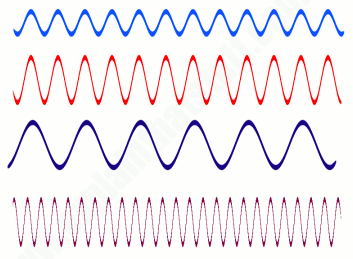# Stationary Process in Time Series

A common assumption made in time series analysis is that one of the components of the pattern exhibited by a time series is the stationary series. This is the random or irregular component we discussed earlier. This random variation is not explained by any other factor.

There are three characteristics of a stationary series:

1. It has a constant mean over time, i.e., the mean does not change with the passage of time.
2. It has a constant variance over time, i.e., the variance/volatility in the data does not change over time.
3. It's autocorrelation remains the same over time. Autocorrelation is a situation in which time series data is influenced by its own historical values. We will learn about this shortly.

The following are all examples of stationary series. They all have constant statistical properties such as mean, variation, autocorrelation, etc.### Why Make Series Stationary?

While performing times series analysis, data scientists will generally make the series stationary. There are two compelling reasons to stationarize a series:

1. Most statistical forecasting models are based on the assumption that the underlying time series can be transformed to a stationary series using mathematical transformations. It is relatively easy to make prediction on a stationary series - the idea being that you can assume that its statistical properties will remain the same in the future as in the past! Once the prediction has been made with the stationary series, we need to untransform the series, that is, we reverse the mathematical transformations we applied to it in the past. This gives us the prediction of the original series. This is generally taken care of by the R software. Therefore for making a good prediction, it is important to find the most suitable sequence of transformations needed to stationarize a time series. This provides important clues in our search for the right forecasting model. Making a series stationary is an important part of fitting an ARIMA model, which we will be learning.
2. Stationarizing a time series also helps us get important sample statistics such as means, variances, and correlations with other variables. As described above, these statistics are useful to predict the future behavior only if the series is stationary. For example, if the series is consistently increasing over time, the sample mean and variance will grow with the size of the sample, and they will always underestimate the mean and variance in future periods. And if the mean and variance of a series are not well-defined, then neither are its correlations with other variables. For this reason you should be cautious about trying to extrapolate regression models fitted to nonstationary data.

What is meant by series is consistently increasing over time?

This was an example of a non-stationary series for which both the mean and covariance are increasing over time. You would observe two trends in such a time series. 1) There will be a clear upward trend, i.e., the mean is increasing over time. 2) the variability of the data is increasing over time. As an example, think of a stock whose price is consistently increasing over time and the volatility of the stock price is also going up with the passage of time.